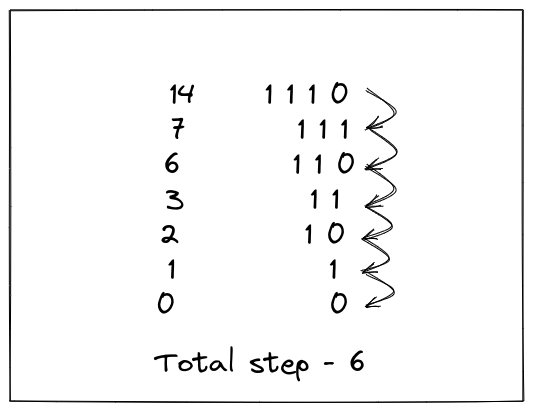Pankaj Tanwar
Published on

# Number of Steps to Reduce a Number to Zero.

––– views
Authors
•Name
Pankaj Tanwar
@the2ndfloorguy

It's day 23 of my coding diary and it really feels amazing.

Problem of the day - Number of Steps to Reduce a Number to Zero

Tag - Easy

Given an integer num, return the number of steps to reduce it to zero.

In one step, if the current number is even, you have to divide it by 2, otherwise, you have to subtract 1 from it.

Example 1:

Input: num = 14 Output: 6

The very obvious approach would be to simply write down a beautiful while loop to iterate till we reach zero.

But I suspect, that's not the intention of the problem. It's something beyond that. Anyway, here is my simple and easy code for the code.

class Solution {public:    int numberOfSteps(int num) {        int res = 0;        while(num > 0) {            if(num % 2 == 0) {                num /= 2;            } else {                num--;            }            res++;        }        return res;    }};

I am not sure, but I feel, there is some interesting logic behind this problem. I again, read the problem statement and tried to figure out the pattern.

Voila....!!!! I think I got this.

If we carefully observe, if a number is divisible by 2, in binary, the last element would be 0 else 1 (so subtracting 1 from the number will again bring it to zero).

Let's take an example of number 14.• Divide from 2 means, shifting right by one.
• Subtracting 1 means, flipping the last 1 to 0.

So, what we can do here is, just count the number of set bits (1 in binary representation) plus, total number of bits in the number minus 1.

result = total set bits + total bits - 1

• Last, 1 is subtracted because we don't need to shift the last 0.

There is a couple of ways find out the number of set bits in a number in C++.

1. __builtin_popcount(num)

2. bitset< 32 >(num).count()

To find out the total number of bits in a number.

Let's utilize it to solve the problem -

class Solution {public:    int numberOfSteps(int num) {        return num == 0 ? 0 : log2(num) + bitset<32>(num).count();    }};

For anyone wondering, the time complexity is O(log n) and space is O(1).

Please do let me know if you have some other approach to share.

Thanks for being part of my daily-code-workout journey. As always, if you have any thoughts about anything shared above, don't hesitate to reach out.

You might like previous editions of my coding diary